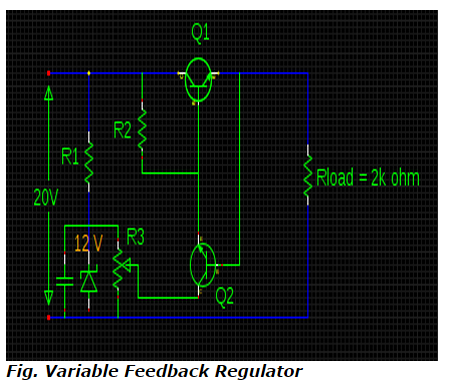# Numerical - VOUT & P1 in a variable feedback regulator circuit

## Compute the values of VOUT & P1 in a variable feedback regulator circuit with Vin = 20V, Vz = 12V & RL = 2K if the transistor is of silicon and the wiper of R3 is adjusted in half-way configuration.a. 16.8 V & 137.76 mW
b. 10.7 V & 80.09 mW
c. 6.7 V & 43.89 mW
d. 4.7 V & 35.79 mW
Correct Answer: c. 6.7 V & 43.89 mW

Explanation

From the configuration of feedback regulator circuit,
VOUT = Voltage at wiper + VBE2

Voltage at wiper must be equal to half the Zener voltage since wiper is adjusted in half way configuration.

VOUT = (12 / 2) + 0.7

= 6.7 V

P1 = VCE1 x IE1

But, VCE1 = Vin - Vout
= 20 – 6.7
= 13.3 V

IE1 = IL = Vout / RL = 6.7 /2k = 3.3 mA

Therefore, P1 = 13.3 x 3.3 = 43.89 mW